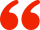# 用Python实现一个基于EG协整法的跨周期套利策略# 一些金融学与数学背景

1987年Engle和Granger提出的协整理论及其方法，为非平稳序列的建模提供了另一种途径。虽然一些经济变量的本身是非平稳序列，但是，它们的线性组合却有可能是平稳序列。这种平稳的线性组合被称为协整方程，且可解释为变量之间的长期稳定的均衡关系。

# 将以上概念运用到期货交易中

statsmodels（http://www.statsmodels.org）是一个Python库，用于拟合多种统计模型，执行统计测试以及数据探索和可视化，是目前计量经济学和数据科学研究中必不可少的神兵利器。它包含很多的经典频率学派统计方法，而且目前人工智能领域中炙手可热的贝叶斯方法和机器学习模型也可在其中找到。

• 线性模型，广义线性模型和鲁棒线性模型

• 线性混合效应模型

• 方差分析（ANOVA）方法

• 时间序列过程和状态空间模型

• 广义的矩量法

# 用Python进行代码实现

``````# 创建一个函数用来协整检验
def cointegration_check(series01, series02):

# 同时平稳或不平稳则差分再次检验
if (urt_rb2001 > 0.1 and urt_rb2005 > 0.1) or (urt_rb2001 < 0.1 and urt_rb2005 < 0.1):

# 同时差分平稳进行OLS回归的残差平稳检验
if urt_diff_rb2001 < 0.1 and urt_diff_rb2005 < 0.1:
matrix = np.vstack([series02, np.ones(len(series02))]).T
beta, c = np.linalg.lstsq(matrix, series01)
resid = series01 - beta * series02 - c
result = 0.0
else:
result = 1.0
return beta, c, resid, result
else:
result = 0.0
return 0.0, 0.0, 0.0, result

else:
result = 0.0
return 0.0, 0.0, 0.0, result
``````

``````# 计算协整
def cointegration_calc():

# 使用发明者量化交易类库
obj = ext.NewPositionManager()

# 展示两个价格序列的协整检验的结果
beta, c, resid, result = cointegration_check(close_01, close_02)

# 如果返回协整检验不通过的结果则全平仓位等待
if not result:
print('协整检验不通过,全平所有仓位')
obj.CoverAll()
return

# 计算残差的标准差上下轨
mean = np.mean(resid)
up = mean + 0.9 * np.std(resid)
down = mean - 0.9 * np.std(resid)

# 计算新残差
resid_new = close_01[-1] - beta * close_02[-1] - c
``````

``````import types
import numpy as np
import statsmodels.tsa.stattools as ts

# 我们首先创建一个函数用来协整检验
def cointegration_check(series01, series02):

# 同时平稳或不平稳则差分再次检验
if (urt_rb2001 > 0.1 and urt_rb2005 > 0.1) or (urt_rb2001 < 0.1 and urt_rb2005 < 0.1):

# 同时差分平稳进行OLS回归的残差平稳检验
if urt_diff_rb2001 < 0.1 and urt_diff_rb2005 < 0.1:
matrix = np.vstack([series02, np.ones(len(series02))]).T
beta, c = np.linalg.lstsq(matrix, series01)
resid = series01 - beta * series02 - c
result = 0.0
else:
result = 1.0
return beta, c, resid, result
else:
result = 0.0
return 0.0, 0.0, 0.0, result

else:
result = 0.0
return 0.0, 0.0, 0.0, result

# 初始化合约数据
def init():

# 订阅螺纹钢的2001合约与2005合约，并且取得发明者量化平台当前周期的所有收盘价
exchange.SetContractType("rb2001")
records = exchange.GetRecords()
close_01 = records.Close

exchange.SetContractType("rb2005")
records = exchange.GetRecords()
close_02 = records.Close

# 计算协整
def cointegration_calc():

# 使用发明者量化交易类库
obj = ext.NewPositionManager()

# 展示两个价格序列的协整检验的结果
beta, c, resid, result = cointegration_check(close_01, close_02)

# 如果返回协整检验不通过的结果则全平仓位等待
if not result:
print('协整检验不通过,全平所有仓位')
obj.CoverAll()
return

# 计算残差的标准差上下轨
mean = np.mean(resid)
up = mean + 0.9 * np.std(resid)
down = mean - 0.9 * np.std(resid)

# 计算新残差
resid_new = close_01[-1] - beta * close_02[-1] - c

obj = ext.NewPositionManager() # 使用发明者量化交易类库

# 此处用来获取持仓信息
positions = exchange.GetPosition() # 获取持仓数组
if len(positions) == 0: # 如果持仓数组的长度是0
return 0 # 证明是空仓，返回0
for i in range(len(positions)): # 遍历持仓数组
if (positions[i]['Type'] == PD_LONG) or (positions[i]['Type'] == PD_LONG_YD):
position_01_long = 1 # 将position_01_long标记为1

elif (positions[i]['Type'] == PD_SHORT) or (positions[i]['Type'] == PD_SHORT_YD):
position_01_short = -1 # 将position_01_short标记为-1

if not position_01_long = 1 and not position_01_short = -1:

# 上穿上轨时做空新残差
if resid_new > up:

obj.OpenLong("rb2001", 1) # 买开
obj.OpenShort("rb2005", 1) # 卖开

# 下穿下轨时做多新残差
if resid_new < down:

obj.OpenLong("rb2005", 1) # 买开
obj.OpenShort("rb2001", 1) # 卖开

# 新残差回归时平仓
elif position_01_short = -1:

if resid_new <= up:
obj.CoverAll()
print('价格回归,平掉所有仓位')

# 突破下轨反向开仓
if resid_new < down:
obj.OpenLong("rb2001", 1) # 买开
obj.OpenShort("rb2005", 1) # 卖开

elif position_01_long = 1:
if resid_new >= down:
obj.CoverAll()
print('价格回归,平所有仓位')

# 突破上轨反向开仓
if resid_new > up:
obj.OpenLong("rb2005", 1) # 买开
obj.OpenShort("rb2001", 1) # 卖开

def main():
while True: